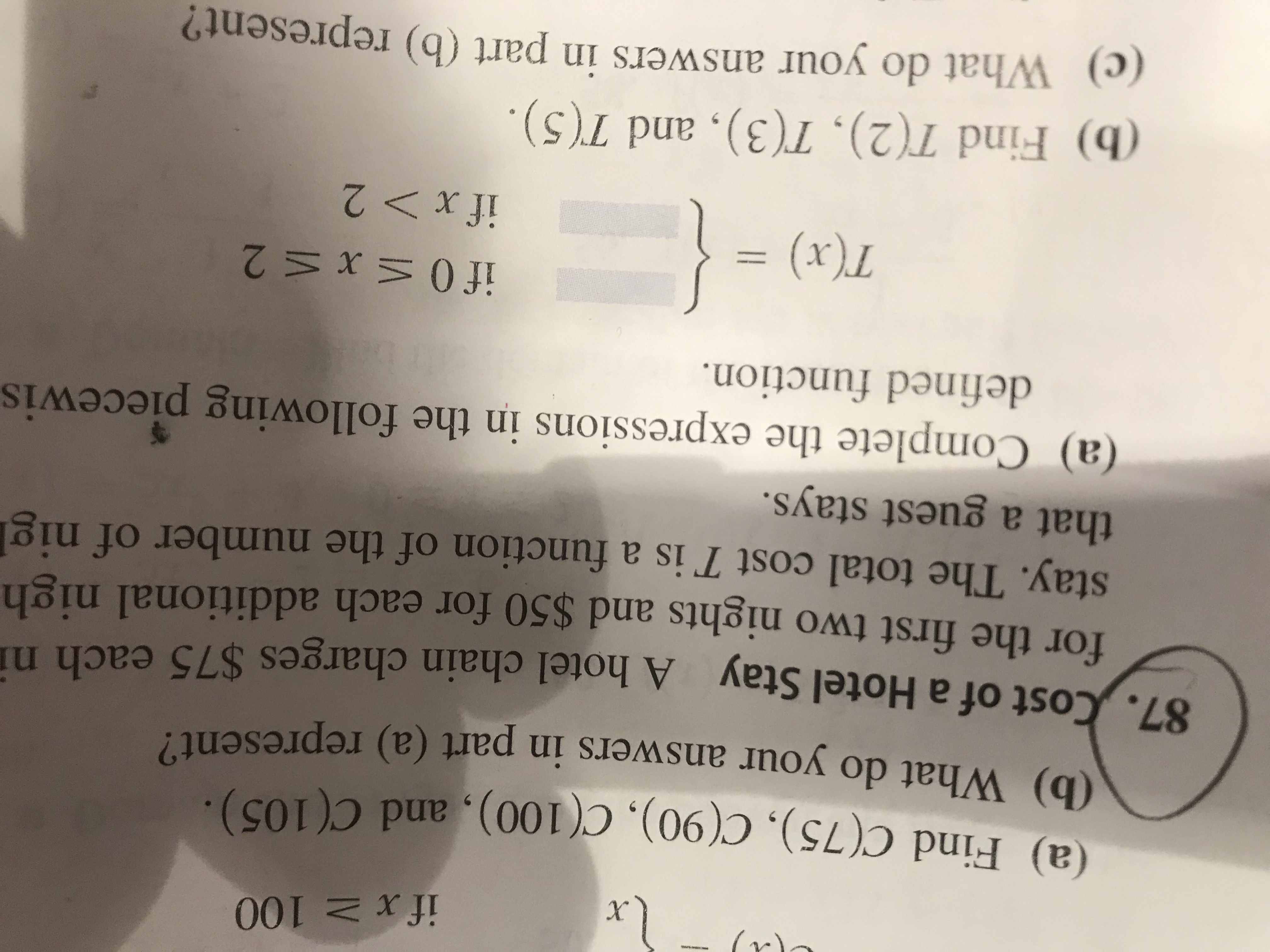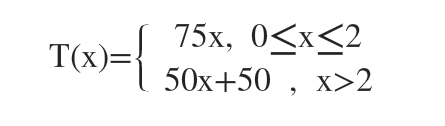# if x > 100x)(a) Find C(75), C(90), C(100), and C(105).(b) What do your answers in part (a) represent?87. Cost of a Hotel Stay A hotel chain charges \$75 each nifor the first two nights and \$50 for each additional nighstay. The total cost T is a function of the number of niglthat a guest stays.87.(a) Complete the expressions in the following piecewisdefined function.if 0 < x < 2= (x),if x > 2}(b) Find T(2), T(3), and T(5).(c) What do your answers in part (b) represent?

Question
11 viewshelp_outlineImage Transcriptioncloseif x > 100 x) (a) Find C(75), C(90), C(100), and C(105). (b) What do your answers in part (a) represent? 87. Cost of a Hotel Stay A hotel chain charges \$75 each ni for the first two nights and \$50 for each additional nigh stay. The total cost T is a function of the number of nigl that a guest stays. 87. (a) Complete the expressions in the following piecewis defined function. if 0 < x < 2 = (x), if x > 2 } (b) Find T(2), T(3), and T(5). (c) What do your answers in part (b) represent? fullscreen
check_circle

star
star
star
star
star
1 Rating
Step 1

1 night cost \$75

2 nights cost = 75*2=150

So, when x<2 then charge for x nights is 75x.

If x is greater than 2 then they charge 50 extra for each night.

So for x>2, cost is: 150+[(x-2)*50] = 150+50x-100=50x+50Step 2

b) T(2)= 75*2=150

T(3)=50(3)+50=200

T(5)=50(5)+50=30...

### Want to see the full answer?

See Solution

#### Want to see this answer and more?

Solutions are written by subject experts who are available 24/7. Questions are typically answered within 1 hour.*

See Solution
*Response times may vary by subject and question.
Tagged in